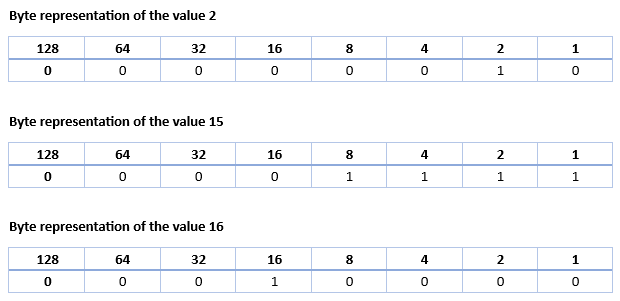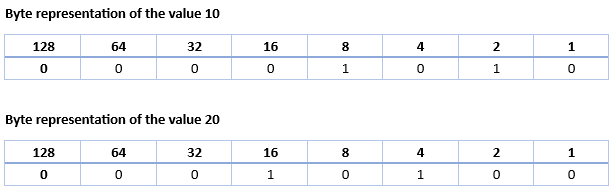# Things in C# you might have missed

## Posted by Filip Ekberg on 18 Sep 2012

A while back I did a short programming quiz on my blog and a lot of you responded to each question with interesting ways to solve those puzzles. Here is a list with those questions among other interesting things that you might now have used in your day-to-day development.

### Bits n' Bytes

The more you know about the internals in the system you are working on, the greater your advantage is. Let's focus on the bits and bytes for now. A bit is either a 1 or a 0 which represents value/no-value or on/off; a byte is a sequence of 8 bits.

Take a look at these three tables:As you might see this is a base-2 representation. It starts at 1, then 2, then 4 and so forth to how far you'd like to go. For simplicity, the table only goes to 128. By letting some of them be 1s and some be 0s, you can represent any number you like.

Fun fact: the ASCII decimal value for the capital letter A is 65 which means the bit-representation would look like this: `01000001` if added to the above table!

Now when we do this, we can do something really powerful called bit-shifting. This means moving the bit `N` steps to either direction (left/right).

This takes us to the first question that I shouted out on twitter:

How can we multiply any given value by 2 without using any arithmetic operations?

The answer is simple, we use bit-shift! Below is an example of how we can do this.

``````int x = 10;
int result = x << 1;
``````

The `<<` means that we shift it to the left and the following value defines how many steps. Below is another table showing what changed, all bits were moved 1 step to the left.There are lot of more interesting things you can do when knowing your bits n' bytes, such as logical and/or.

### A number, but not really

Almost 3 years ago I answered a question on StackOverflow where the question was the following:

Suppose you have this loop definition: `while (i == i) ;` What is the type of i and the value of i if the loop is not an infinite loop and the program is using only one thread?

Let us assume that this is built in types in .NET and that we are not allowed to override the equality operators. This makes it pretty hard to figure out, right?

This introduces NaN -- Not a Number. But how can it be a number but not a number at the same time? NaN was introduced because of imaginary numbers such as `Sqrt(-1)`

So, let us try to get the square root of -1 in C#:

``````var result = Math.Sqrt(-1);
Console.WriteLine(result == result);
``````

Can you guess what this prints?

It actually prints false! This is because `NaN == NaN` will always return false! Because comparing something that is not correct with something else that is not correct doesn't make any sense.

But what type is it?

We know that `Math.Sqrt` returns a `double`. So the answer is `Double.NaN`.

### Cleaner code without temporary lists

Do you find yourself having tons of methods that contain temporary lists that you want to return in the end of the method?

For simplicity I'm going to use a method in the following example that I would normally just use LINQ to achieve.

Here I have a method that just check is a list of names contain my pattern:

``````IEnumerable<string> _names = new[] {"Filip", "Sofie"};
IEnumerable<string> GetList(string pattern)
{
var found = new List<string>();

foreach(var name in _names)
{
if(name.Contains(pattern))
{
}
}

return found;
}
``````

It's not really that messy, but it can be cleaned up. If we have a lot of methods like this, we can make the code base much easier to read through. What we can do is to make use of the `yield` keyword like this:

``````IEnumerable<string> _names = new[] {"Filip", "Sofie"};
IEnumerable<string> GetList(string pattern)
{
foreach(var name in _names)
{
if(name.Contains(pattern))
{
yield return name;
}
}
}
``````

### Why are there uppercase and lowercase versions of object/string etc?

`object`, `string`, `int`, `double` and the other value types are just keyword aliases for their representations in `System.*`.

For example, `object` is really `System.Object`, `string` is `System.String` and `int` is `System.Int32`.

When the code is compiled, it doesn't matter if you wrote `string` or `System.String`, it's going to be the same IL!